IFT Notes for Level I CFA® Program
IFT Notes for Level I CFA® Program

# Part 2

## 5. Measures of Central Tendency

### 5.1 The Arithmetic Mean

The arithmetic mean is the sum of the observations divided by the number of observations. It is the most frequently used measure of the middle or center of data.

The Population Mean

The population mean is the arithmetic mean computed for a population. It is expressed as: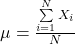where:

N is the number of observations in the entire population and

Xi is the ith observation

Consider the stock returns of a company over the last 10 years: 2%, 5%, 4%, 7%, 8%, 8%, 12%, 10%, 8%, and 5%.

For this data set, the population mean can be computed as: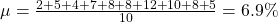The Sample Mean

The sample mean is calculated like the population mean, except that we use the sample values. It is expressed as: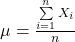where: n is the number of observations in the sample.

If the sample data is: 8, 12, 10, 8 and 5, the sample mean can be calculated as: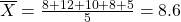### 5.2 The Median

The median is the midpoint of a data set that has been sorted into ascending or descending order.

For odd number of observations: 2,5,7,11,14 → Median = 7

For even number of observations: 3, 9, 10, 20 → Median = (9 + 10)/2 = 9.5

As compared to a mean, a median is less affected by extreme values (outliers).

### 5.3 The Mode

The mode is the most frequently occurring value in a distribution.

For the following data set: 2, 4, 5, 5, 7, 8, 8, 8, 10, 12 → Mode = 8

A distribution can have more than one mode, or even no mode. When a distribution has one mode it is said to be unimodal. If a distribution has two or three modes, it is called bimodal or trimodal respectively.

### 5.4 Other Concepts of Mean

The Weighted Mean

Here different observations are given different proportional influence on the mean. The formula for the weighted mean is: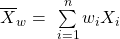where: the sum of the weights equals 1; that is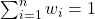Example

Consider an investor with a portfolio of three stocks. $40 is invested in A,$60 in B, and $100 in C. If returns were 5% on A, 7% on B, and 9% on C, compute the portfolio return using the weighted mean. Solution: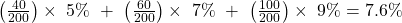An arithmetic mean is a special case of a weighted mean where all observations are equally weighted by the factor 1/n. The Geometric Mean The most common application of the geometric mean is to calculate the average return of an investment. The formula is: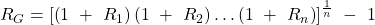Example The return over the last four periods for a given stock is: 10%, 8%, -5% and 2%. Calculate the geometric mean. Solution: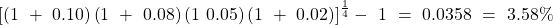Given the returns shown above,$1 invested at the start of period 1 grew to: $1.00 x 1.10 x 1.08 x 0.95 x 1.02 =$1.151. If the investment had grown at 3.58% every period, $1.00 invested at the start of period 1 would have increased to:$1.00 x 1.0358 x 1.0358 x 1.0358 x 1.0358 =$1.151. As expected, both scenarios give the same answer. 3.58% is simply the average growth rate per period. Instructor’s Note In the reading on Discounted Cash Flow Applications, we used the geometric mean to calculate the time-weighted rate of return. The Harmonic Mean The harmonic mean is a special type of weighted mean in which an observation’s weight is inversely proportional to its magnitude. The formula for a harmonic mean is: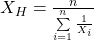where: Xi > 0 for i = 1, 2 … n, and n is the number of observations The harmonic mean is used to find average purchase price for equal periodic investments. Example An investor purchases$1,000 of a security each month for three months. The share prices are $10,$15 and $20 at the three purchase dates. Calculate the average purchase price per share for the security purchased. Solution: The average purchase price is simply the harmonic mean of$10, $15 and$20.

The harmonic mean is: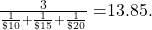A more intuitive way of solving this is total money spent purchasing the shares divided by the total number of shares purchased.

Total money spent purchasing the shares = $1,000 x 3 =$3,000

Total shares purchased = sum of shares bought each month

=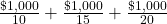=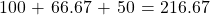Average purchase price per share =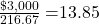Comparison of AM, GM and HM

• If the returns are constant over time: AM = GM = HM.
• If the returns are variable: AM > GM > HM.
• The greater the variability of returns over time, the more the arithmetic mean will exceed the geometric mean.

## 6. Other Measures of Location: Quantiles

A quantile is a value at or below which a stated fraction of the data lies. Some examples of quantiles include:

• Quartiles: The distribution is divided into quarters.
• Quintiles: The distribution is divided into fifths.
• Deciles: The distribution is divided into tenths.
• Percentile: The distribution is divided into hundredths.

The formula for the position of a percentile in a data set with n observations sorted in ascending order is: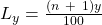where:
y is the percentage point at which we are dividing the distribution.
n is the number of observations.
Ly is the location (L) of the percentile (Py) in an array sorted in ascending order.

Some important points to remember are:

• When the location, Ly, is a whole number, the location corresponds to an actual observation.
• When Ly is not a whole number or integer, Ly lies between the two closest integer numbers (one above and one below) and we use linear interpolation between those two places to determine Py.

Example

Consider the data set:

47        35        37        32        40        39        36        34        35        31       44

1. Find the 75th percentile point
2. Find the 1st quartile point
3. Find the 5th decile point
4. Find the 6th decile point.

Solution to 1:

First arrange the data in ascending order:

31, 32, 34, 35, 35, 36, 37, 39, 40, 44, 47

Location of the 75th percentile is the:
L75 = (11 + 1) (75/100) = 9th value. i.e. P75 = 40

With a small data set, such as this one, the location and the value is approximate. As the data set becomes larger, the location and percentile value estimates become more precise.

Solution to 2:

Location of the 1st quartile is the:

L25 = (11 + 1) (25/100) = 3rd value. i.e. P25 = 34

Solution to 3:

Location of the 5th decile is the:

L50 = (11 + 1) (50/100) = 6th value. i.e. P50 = 36

Solution to 4:

L60 = (11 + 1) (60/100) = 7.2

Use linear interpolation, which estimates an unknown value on the basis of two known values that surround it.

In this case, the 7th value is 37 and the 8th value is 39. The 6th decile is: P60 = 37+ 0.4 (0.2 times the linear distance between 37 and 39). P60 = 37.4